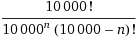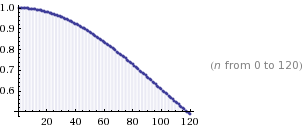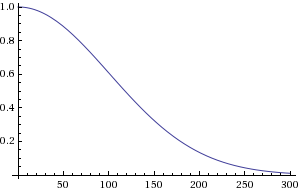# grack.com

## Code

After receiving my Google Voice invite tonight and picking a phone number, seemingly at random, I discovered that I had picked a number with the same last four digits as one of my friends’ numbers.  If you are familiar with the Birthday Paradox, you might recognize the form of the problem.

So, what are the chances that given a number of friends, n, you don’t pick a number that ends in the last four digits as the number of another friend? Well, if it’s just you, the probability that you pick a number that noone else has is 1. With a single friend, the chances that you pick a safe number are 9999/10000. With two friends, the chances are (9998/10000) * (9999/10000), modeling it as on trial after another.

It turns out that you can expand this sequence out and wrap it up in a nice factorial equation. I won’t bore you with the details- it’s idential to the technique used on the wikipedia entry. You’ll end up with the following equation:While having the equation on hand is nice, computing BigInt-magnitude factorials is outside Google’s math evaluation query and Apple’s Calculator application. Fortunately, Wolfram Alpha comes to the rescue (the first time I’ve been able to use it for a real question!):

Enter “10000! / (10000^n * (10000 - n)!)” and you’ll get a detailed analysis of the equation, along with a pretty graph:After some research, I figured out how to limit the plot to get a better idea by using the “from” keyword: 10000! / (10000^n * (10000 - n)!) from n=0 to 300:So, as you can see, if you’ve got 120 friends, your chances are pretty much 50/50 that you’ll have the same last four digits as one of them.